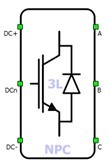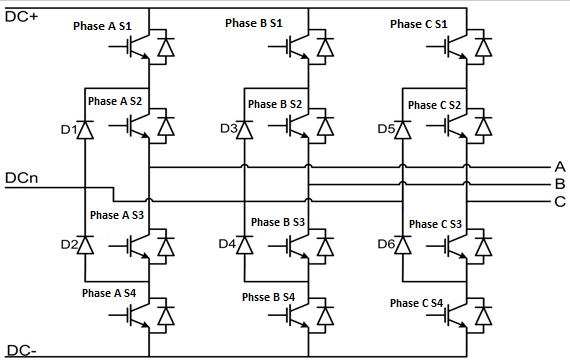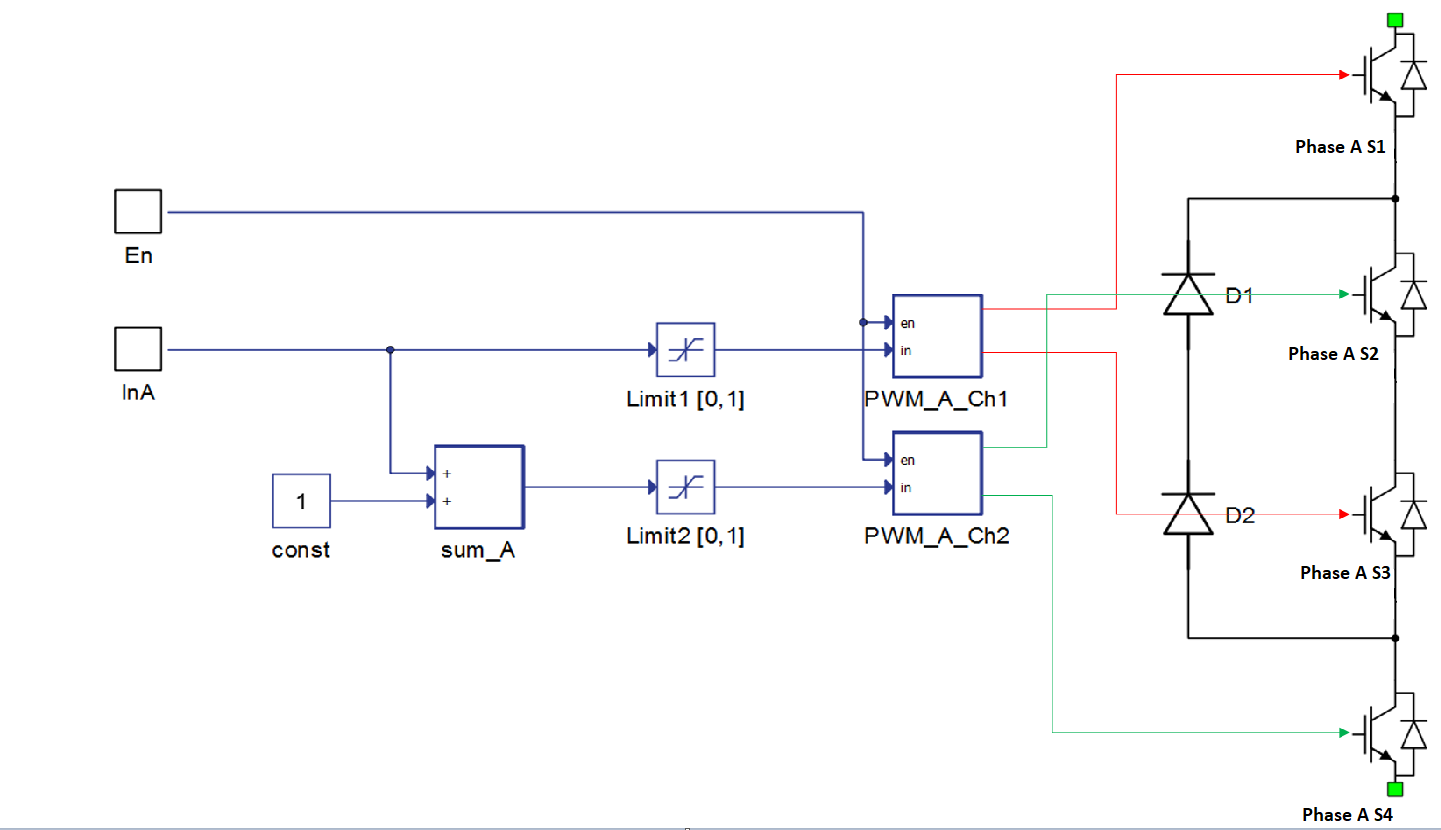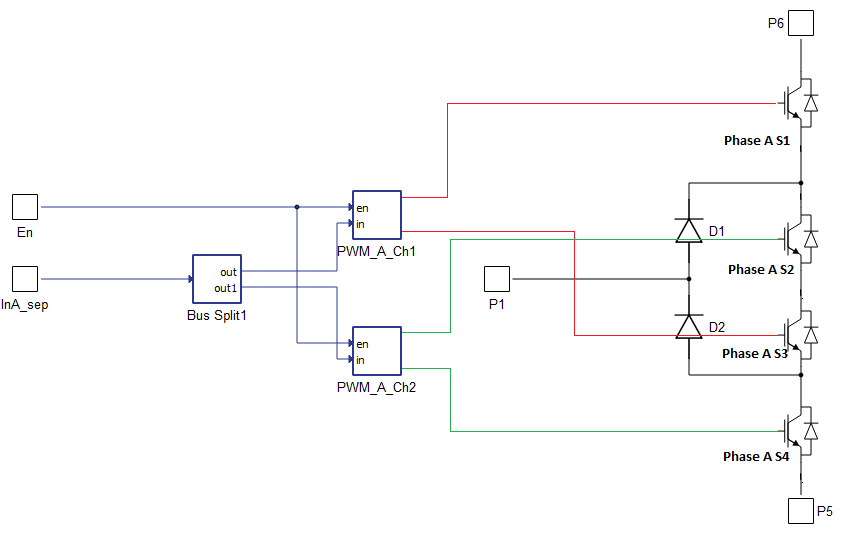# Three Phase NPC Inverter

This section describes three-phase three-level NPC inverters/rectifier

A block diagram and input parameters for this inverter-rectifier block are given in Table 1.

Table 1. Three-phase three-level NPC inverter block in the Schematic Editor core library
component component dialog window component parametersThree phase three level NPC inverter• General
• Control (Digital inputs, Internal modulator, Internal modulator separated, Model)
• Phase A S1 (1..32)
• Phase A S2 (1..32)
• Phase A S3 (1..32)
• Phase A S4 (1..32)
• Phase B S1 (1..32)
• Phase B S2 (1..32)
• Phase B S3 (1..32)
• Phase B S4 (1..32)
• Phase C S1 (1..32)
• Phase C S2 (1..32)
• Phase C S3 (1..32)
• Phase C S4 (1..32)
• gate_logic (active high, active low)
• PWM Switching enabled (enables usage of external digital signal to enable the switching)
• Sen (digital input selection for PWM enable)
• DTV detection
Weight = 3

A schematic block diagram of the inverter switching block is given in Figure 1 with corresponding switch arrangement and naming.

Digital inputs, when selected as the Control parameter, enables you to assign gate drive inputs to any of the digital input pins (from 1 to 32). For example, if Phase A S1 is assigned to 1, the digital input pin 1 will be routed to the Phase A S1 switch gate drive. In addition, the gate_logic parameter selects either active high (i.e. high-level input voltage VIH turns on the switch), or active low (i.e. low-level input voltage VIL turns on the switch) gate drive logic, depending on your external controller design.Figure 1. A schematic block diagram of a three-phase three-level NPC inverter/rectifier block diagram with corresponding switch naming

Internal modulator, when selected as the Control parameter, enables you to use internal PWM modulator for driving the converter's switches instead of digital input pins. In this configuration, four additional component inputs will be present. En input is used to enable/disable PWM modulator control, while InA, InB, and InC are used as referent signal inputs for the internal PWM modulator. Overall, 6 PWM channels are used to drive the three level three phase NPC converter, 2 per phase. Reference signals for 2 modulators that control the switches of a single phase leg, are created from a single reference input. A block diagram of one phase leg controlled by PWM modulators is shown in Figure 2. The input reference signal is in range from -1.0 to 1.0. This signal is split into two reference signals for two modulators, and set to a range from 0.0 to 1.0.Figure 2. Block diagram of a PWM control unit for NPC phase leg

Internal modulator separated, when selected as the Control parameter, enables you to use the internal PWM modulator for driving the converter's switches instead of digital input pins. Additional component inputs and the number of created internal PWM modulators are the same as described in the Internal modulator section. Reference signals for 2 modulators that control the switches of a single phase leg are provided to the component through a vectorized input (i.e. the value on index 0 controls the PWM_A_Ch1 modulator, while the value on index 1 controls the PWM_A_Ch2 modulator). A block diagram of one phase leg separately controlled by PWM modulators is shown in Figure 3. The input reference signal range is adjustable from the component properties.Figure 3. Block diagram of the separated control method for NPC phase leg

Model, when selected as the Control parameter, enables you to set the IGBT gate drive signals directly from the signal processing model. The input pin gates appears on the component and requires a vector input of twelve gate drive signals in the following order: [Phase A S1, Phase A S2,…, Phase B S1, Phase B S2,…, Phase C S1, Phase C S2,…]. When controlled from the model, the logic is always set to active high.

PWM Switching enabled, when selected, enables use of an external PWM enabling digital signal.

DTV detection, when selected, causes DTV detection to be signalized during simulation runtime.

## Timing

When Enable delays is enabled, the turn on and turn off delay of the IGBTs will be included in the simulation. More information about this feature can be found in the dedicated switching delay section.

## Losses calculation

When the Losses calculation property is enabled, the component will calculate switching and conduction power losses for all switching elements (IGBTs and Diodes or MOSFETs). For MOSFET switching elements, the diode characteristic represents the internal MOSFET body diode. Switching power losses are calculated as a function of current, voltage, and temperature using 3D lookup tables. Also, 2D input for losses is supported. When a 2D losses table is inserted, it assumes only current (I) and temperature dependence. From version 2020.3, conduction power losses can be defined as a function of current and temperature using Vt and Vd lookup tables (LUTs) (the previous option of using Vce, Rce, Vd, and Rd properties are removed). These LUTs can be 1D or 2D tables. If the LUT is a 1D table, forward voltage drop depends only on current, but if LUT is a 2D table, forward voltage drop dependence on the junction temperature is also considered. In the MOSFET case under reverse current conduction, a current sharing calculation between the MOSFET channel and the internal body diode is performed. Import options and an explanation how to correctly fill all necessary power losses parameters is described in the import power losses section.

For the IGBT switch type, for both Losses groups, input/output terminals for power losses receive/generates vectors of 30 elements (every index in the vector represent one switching element). The first 10 elements (indexes 0-9) are related to the switching elements in phase A, the second 10 elements (indexes 10-19) are related to the switching elements in phase B, and the last 10 elements (indexes 20-29) are related to the switching elements in phase C. For the MOSFET switch type, for both Losses groups, input/output terminals for power losses receive/generate vectors of 18 elements (every index in the vector represent one switching element). The first 6 elements (indexes 0-5) are related to the switching elements in phase A, the second 6 elements (indexes 6-11) are related to the switching elements in phase B, and the last 6 elements (indexes 12-17) are related to the switching elements in phase C. If different switch types are used for the Losses groups, input/output terminals for power losses receive/generate vectors of 24 elements, with the same ordering logic as previously described. Switching element ordering and group definition for each phase are described in the NPC Leg Losses calculation section. All switches are distributed in three groups, and for each group, different power loss parameters can be specified (Phase A S1, Phase A S4, Phase B S1, Phase B S4, Phase C S1, and Phase C S4 are in group 1; Phase A S2, Phase A S3, Phase B S2, Phase B S3, Phase C S2, and Phase C S3 are in group 2; D1, D2, D3, D4, D5, and D6 are in group 3). Available component properties are:
• Losses groups - Switching elements group
• Current values - Switching elements current axis [A]

• Voltage values - Switching elements voltage axis [V]

• Temp values - Switching elements temperature axis [°C]

• Vce - IGBT collector emitter saturation voltage [V] - discontinued from 2020.3 release

• Rce - IGBT on state slope resistance [Ohm] - discontinued from 2020.3 release

• Vd - IGBT Diode voltage drop [V] - discontinued from 2020.3 release

• Rd - IGBT Diode slope resistance [Ohm] - discontinued from 2020.3 release

• Vt table - Switch forward voltage drop, f(I,T) [V]

• Vd table - Diode forward voltage drop, f(I,T) [V]

• Et on table - Switch switching ON losses, output energy, f(I, V, T) [J]

• Et off table - Switch switching OFF losses, output energy, f(I, V, T) [J]

• Ed off table - Diode switching OFF losses, output energy, f(I, V, T) [J]

Note: Automatic initialization of the Vt table and Vd table properties will be performed based on the discontinued Voltage and Resistance properties (Vce, Rce, Vd, Rd).

## Temperatures calculation

When the Temperatures calculation property is enabled, the component will calculate the combined power losses (P_loss) and junction temperatures (T_junctions) for all switching elements (IGBTs and diodes). Combined power losses represent sum of the calculated switching and conduction losses transferred through the internally generated Thermal network component. This Thermal network component also calculates the junction temperatures from power losses, input case temperatures, and provided thermal model parameters. For each switching group different thermal parameters can be specified. Input/output ports for temperature calculation are vectors of 30 elements and they are indexed in the same way as explained in the Losses calculation section. Additional temperature calculation mask properties are:
• Thermal networks type - Defines type of internal thermal network
• Rth switch - List of thermal resistance for the IGBT switch
• Tth switch / Cth switch - List of thermal time constants or thermal capacitances for the IGBT switch
• Rth diode - List of thermal resistance for diode
• Tth diode / Cth diode - List of thermal time constants or thermal capacitances for diode
• Calculations execution rate - Execution rate in [s] for the losses and temperatures calculation logic

## Digital Alias

If a converter is controlled by digital inputs, an alias for every digital input used by the converter will be created. Digital input aliases will be available under the Digital inputs list alongside existing Digital input signals. The alias will be shown as Converter_name.Switch_name, where Converter_name is name of the converter component and Switch_name is name of the controllable switch in the converter.